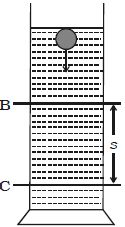# Experimental Determination of Viscosity of Highly Viscous Liquids

The coefficient of highly viscous liquid like castor oil can be determined by Stoke’s method. The experimental liquid is taken in a tall, wide jar. Two marking B and C are marked as shown in Figure. A steel ball is gently dropped in the jar.The marking B is made well below the free surface of the liquid so that by the time ball reaches B, it would have acquired terminal velocity v.

When the ball crosses B, a stopwatch is switched on and the time taken t to reach C is noted. If the distance BC is s, then terminal velocity v = S/t

The expression for terminal velocity is: v = 2/9 [a2 (ρ-σ)g]/η

So, S/t = 2/9 [a2 (ρ-σ)g]/η

Or, η = 2/9 a2 (ρ-σ)g (t/S)

Knowing a, ρ and σ, the value of η of the liquid is determined.